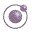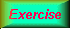1 minute, 32 seconds Higher-order Functions - slide 4 : 34

Linear search in lists
Search criterias can be passed as predicates to linear search functions
```;; A simple linear list search function.
;; Return the first element which satisfies the predicate pred.
;; If no such element is found, return #f.
(define (find-in-list pred lst)
(cond ((null? lst) #f)
((pred (car lst)) (car lst))
(else (find-in-list pred (cdr lst)))))```A sample interaction using find-in-list.Linear string searchIndex in listBinary search in sorted vectors